# AP Physics 1 Multiple-Choice Practice Test 35

### Test Information10 questions18 minutes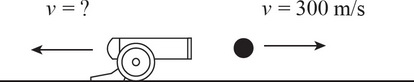1. A soldier loads a 10 kg cannonball into a 300 kg cannon that is initially at rest on the ground. What is the recoil speed of the cannon if the cannonball is fired with a horizontal velocity of 300 m/s ?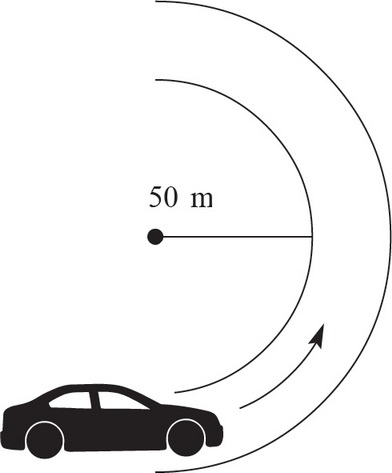2. A car is driving on a flat curve with a radius of 50 m. If the coefficient of friction between the ground and the car's tires is 0.8, what is the maximum speed of the car in order to make the curve without sliding?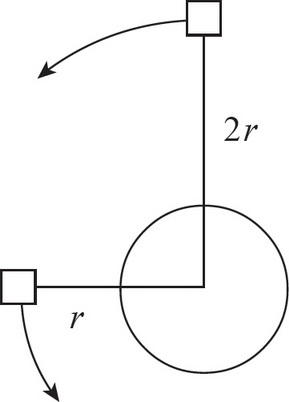3. If the speed of a satellite orbiting the Earth at a distance r from the center of the Earth is v, what is the speed of a second satellite orbiting the Earth at a distance 2r from the center of the Earth?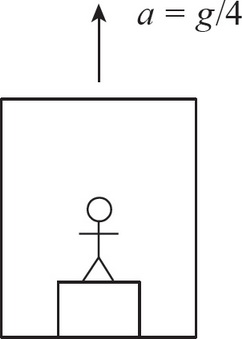4. A 50 kg man stands on a scale that measures force in an elevator traveling up with an acceleration of g/4. What will the scale read?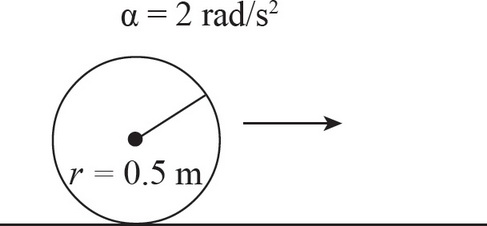5. A mechanical wheel initially at rest on the floor begins rolling forward with an angular acceleration of 2 rad/s2. If the radius of the wheel is 0.5 m, what is the linear velocity of the wheel after 5 s ?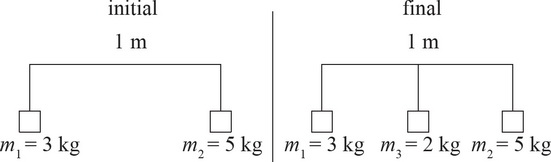6. Two masses are attached to a 1 m long massless bar. Mass 1 is 3 kg and is attached to the far left side of the bar. Mass 2 is 5 kg and is attached to the far right side of the bar. If a third mass that is 2 kg is added to the middle of the bar, how does the center of mass of the system change?

7. A 5 kg box is connected to a pulley with rope in the diagram shown below. If the radius of the pulley is 0.5 m, what is the torque generated by the box on the pulley?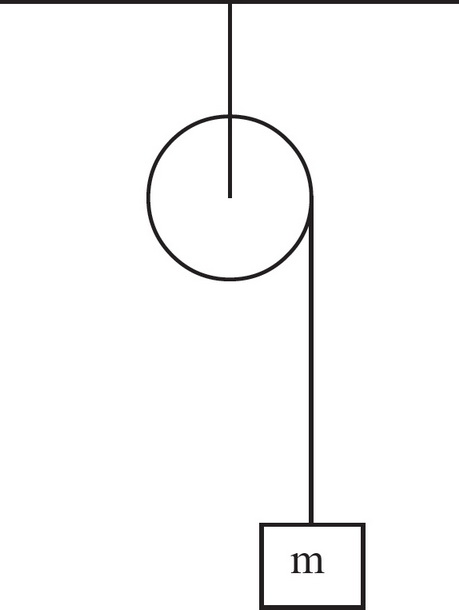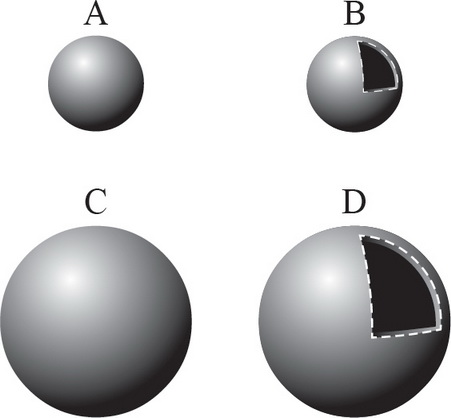8. Which of the following objects has the greatest rotational inertia?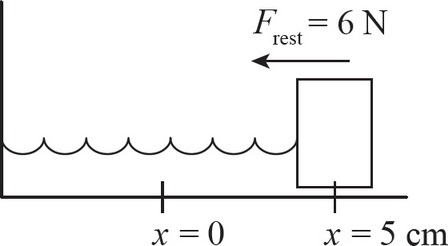9. A horizontal spring is attached to a 5 kg block. When the block is pulled 5 cm to the right, the restoring force has a magnitude of 6 N. What is the frequency of the spring?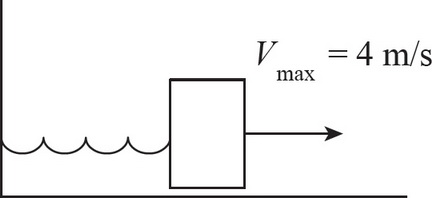10. A 3 kg block is attached to a horizontal spring with a force constant of 10 N/m. If the maximum speed of the block is 4 m/s, what is the amplitude of the block?